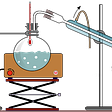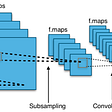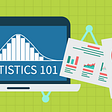# Introduction:

Linear Regression is usually the first machine learning algorithm. It is a simple model but everyone needs to master it as it lays the foundation for other machine learning algorithms.

To understand the linear regression, lets first understand what is regression.

It is basically statistical approach to find the relationship between variables/features. They are used to predict continuous values.

# Intuition :

Linear Regression is supervised machine learning algorithm.It establishes a relationship between dependent variable (Y) and one or more independent variables (X) using a best fit straight line (also known as regression line).

Dependent Variable : Variable for which we want to predict value also called target viables.

Independent Variable: Variable which is used to predict the values

Where :

Area(in sft) : Independent variable

Price : Dependent Variable

In two-dimensional space we create a line to find the relationship between both the variables.

Equation of line:

y= m*x + c

Where :

y = Dependent Variable

x = Independent Variable

m = Slope which indicate that within the unit change in value of x how much y is changing.

c = intercept or bias which says that data is not starting from zero.

Our target is to find best fit line which can be used to predict the dependent values(here it is denoted as y).

But there can be multiple lines in the two-dimensional space so which line is the best fit line ?

To understand this we should understand Residual Sum of Squares/Sum of squared errors(RSS/SSE).

Residual sum of squares (also known as sum of squared errors of prediction) is the difference between actual data points and predicted data points.

Equation of SSE:

Where

yi= Observer data Points

ŷi: Actual value estimated by regression line.

Using SSE/RSS equation ,Multiple lines will be created as shown below.

Line which will have least RSS/SSE value is selected as best fit line for regression in two-dimensional space. Basically that line should be considered which is covering most of the point and having least RSS/SSE Values.

Here

Blue Dots : Actual Data Point,Red Line: Predicted Data point

It is to be noted that we are taking only two variables/features for ease of demonstration.

In case of 2 variables : We can draw a line

3 Variables : Plane can be drawn

4 Variables : Hyperplane can be drawn

Similarly independent feature with N dimension ,a relative N-Dimension space is created(Since it’s difficult to show higher dimension I am taking example with 2 dimension)

Above line of equation can also be also written as below

Y=Wo+W1*X1

Let’s put our Y value in the RSS/SSE equation:

RSS(Wo,W1) = (y1- [Wo+W1*X1]) ² + (y2-[ Wo+W1*X2]) ²………+(yi-[Wo+W1*Xi]) ²

Finally all the values of independent variables are passed to this equation along with there weights(Intercept and Slope values) and line which is having least Residual sum of squares are selected as best fit line.

To update weights we use Cost Function(also called squared error function discussed above) and Gradient Descent which we will discuss in our later topics.To Give you glimpse.

A Cost Function basically tell us ‘How Good’ our model is at making the prediction for a given value of m(Wi) and c(Wo).

Cost Function is denoted as J.

Our target is to minimize the cost function.To do this we need to find that value of m(or w1) that produce lowest value of Y.

Gradient descent can be used to minimize the cost function.Gradient descent algorithm helps to make this decision with the use of Derivatives.

Using gradient descent we will be able to find the those values of m(slope) and b(intercept) for which our cost function is minimum.Please go through below article to get in depth details about Gradient Descent.

Best Practices to be considered before making the linear regression model:

1. Since it uses distance(mostly euclidean distance)as a metrics to find actual and predicted values,Standardization/Normalization is must before using data into model training.Standardization (generally it is better as it take cares of outliers)converts data into standard normal distribution or z-distribution where mean is 0 and standard deviation is 1.Basically it will make data unit less.

Z-Score is used to perform standardization.

Where as goal of normalization is to change the values of numeric columns in the dataset to a common scale, without distorting differences in the ranges of values.

2. Remove the outliers for linear regression.You can check outliers with five number summary or by using box plot or scatter plot.

3.Remove Correlated(features which are related to each other such that increase of one feature, increases or decreases other) Features.

Model Fitness and Accuracy :

1. Overfitting happens when training and test data has huge difference in accuracy. Preprocessing and feature engineering should be done to get rid of over fitting and get good model accuracy.
2. In linear Regression ,Model accuracy is determined by the value of R-Squared.Statistically R-Squared is a measure of ,how close the data are to the fitted regression line.This is also known as coefficient of determination.

R-squared = Explained variation / Total variation

R-squared value always lies between 0 and 100% :

• 0% indicates that the model explains none of the variability of the response data around its mean.
• 100% indicates that the model explains all the variability of the response data around its mean.

In general, the higher the R-squared, the better the model fits your data.

Conclusion : Linear Regression is one of the basic models which is used to predict continuous values.Data Preprocessing and Feature engineering is must before creating any Linear regression model to get good accuracy.

Please hit Clap(up to 50 claps) if you like my article,which will motivate me for writing more.

Want to connect :

If you like my posts here on Medium and would wish for me to continue doing this work, consider supporting me on patreon

--

--

--

## More from Analytics Vidhya

Analytics Vidhya is a community of Analytics and Data Science professionals. We are building the next-gen data science ecosystem https://www.analyticsvidhya.com

## Day 150 of #NLP365: NLP Papers Summary — Will-They-Won’t-They: A Very Large Dataset for Stance…## Sigmoid Activation and Binary Crossentropy — A Less Than Perfect Match?## Knowledge Distillation — Study Notes## Deploying a Machine Learning Model Using a Flask Application + API## An Overview of ML on AWS## Convolution Operation in CNN:## Creating a perceptron network: from zero to hero## Anjani Kumar

Data Science ,ML & NLP Enthusiastic

## Statistics for Data Science## Deep dive of Regularizations techniques## Checking your linear regression assumptions and how to check them## A Guide to Model Selection For Survival Analysis